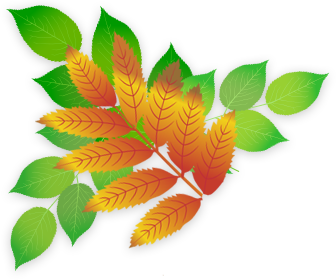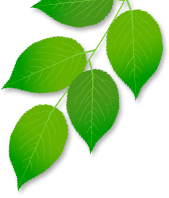## Developing Numeracy In Year 1

As they move out of Reception, children in Year 1 begin to study the National Curriculum for Mathematics. They continue to work with practical apparatus and real objects (called 'manipulatives'). to help them visualise concepts such as number bonds (3+7=10, 13+7-=20). They start to look at telling the time and calculate amounts of money. The ideas of multiplication and division are developed through grouping and sharing.

These are the objectives that children will be taught in Numeracy during Year 1.

• count to and across 100, forwards and backwards, beginning with 0 or 1, or from any given number
• count, read and write numbers to 100 in numerals; count in multiples of twos, fives and tens
• given a number, identify one more and one less
• identify and represent numbers using objects and pictorial representations including the number line, and use the language of: equal to, more than, less than (fewer), most, least
• read and write numbers from 1 to 20 in numerals and words.

• read, write and interpret mathematical statements involving addition (+), subtraction (–) and equals (=) signs
• represent and use number bonds and related subtraction facts within 20
• add and subtract one-digit and two-digit numbers to 20, including zero
• solve one-step problems that involve addition and subtraction, using concrete objects and pictorial representations, and missing number problems such as 7 = â˜ – 9.

Year 1: Multiplication and Division

• solve one-step problems involving multiplication and division, by calculating the answer using concrete objects, pictorial representations and arrays with the support of the teacher.

Year 1: Fractions (including decimals and percentages)

• recognise, find and name a half as one of two equal parts of an object, shape or quantity
• recognise, find and name a quarter as one of four equal parts of an object, shape or quantity

Year 1: Measurement

• compare, describe and solve practical problems for:
• lengths and heights [for example, long/short, longer/shorter, tall/short, double/half]
• mass/weight [for example, heavy/light, heavier than, lighter than]
• capacity and volume [for example, full/empty, more than, less than, half, half full, quarter]
• time [for example, quicker, slower, earlier, later]
• measure and begin to record the following:
• lengths and heights
• mass/weight
• capacity and volume
• time (hours, minutes, seconds)
• recognise and know the value of different denominations of coins and notes
• sequence events in chronological order using language [for example, before and after, next, first, today, yesterday, tomorrow, morning, afternoon and evening]
• recognise and use language relating to dates, including days of the week, weeks, months and years
• tell the time to the hour and half past the hour and draw the hands on a clock face to show these time

Year 1: Geometry - properties of shapes

• recognise and name common 2-D and 3-D shapes, including:
• 2-D shapes [for example, rectangles (including squares), circles and triangles]
• 3-D shapes [for example, cuboids (including cubes), pyramids and spheres].